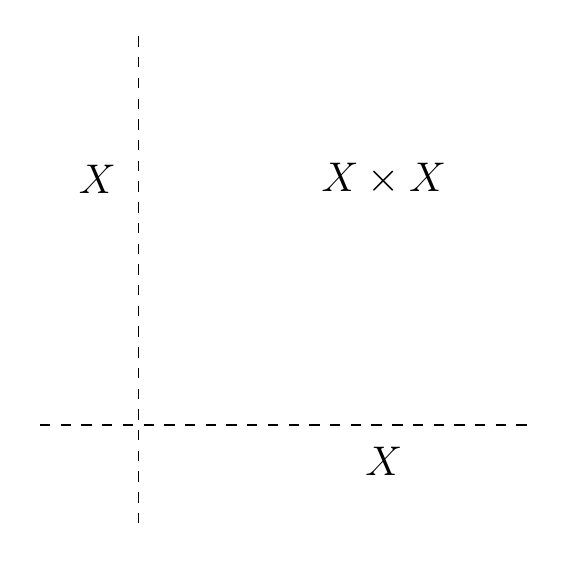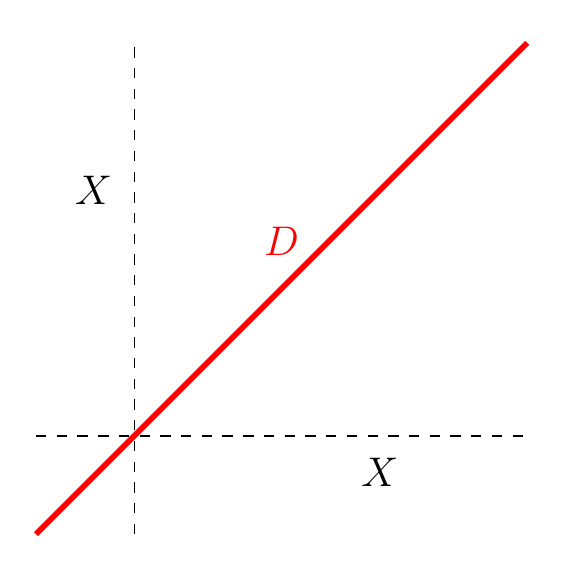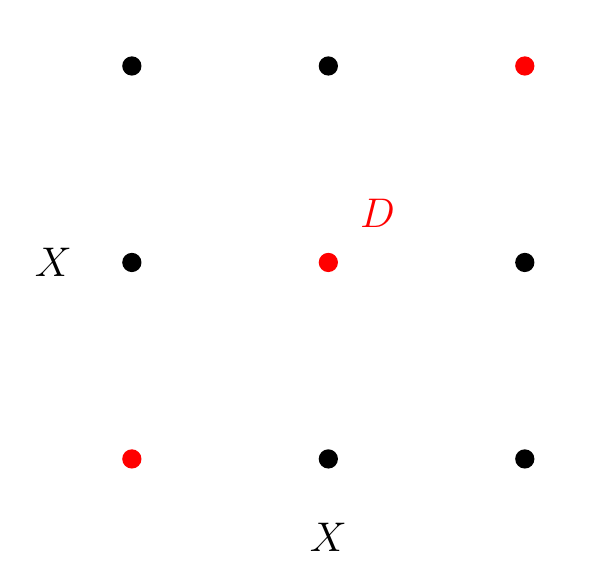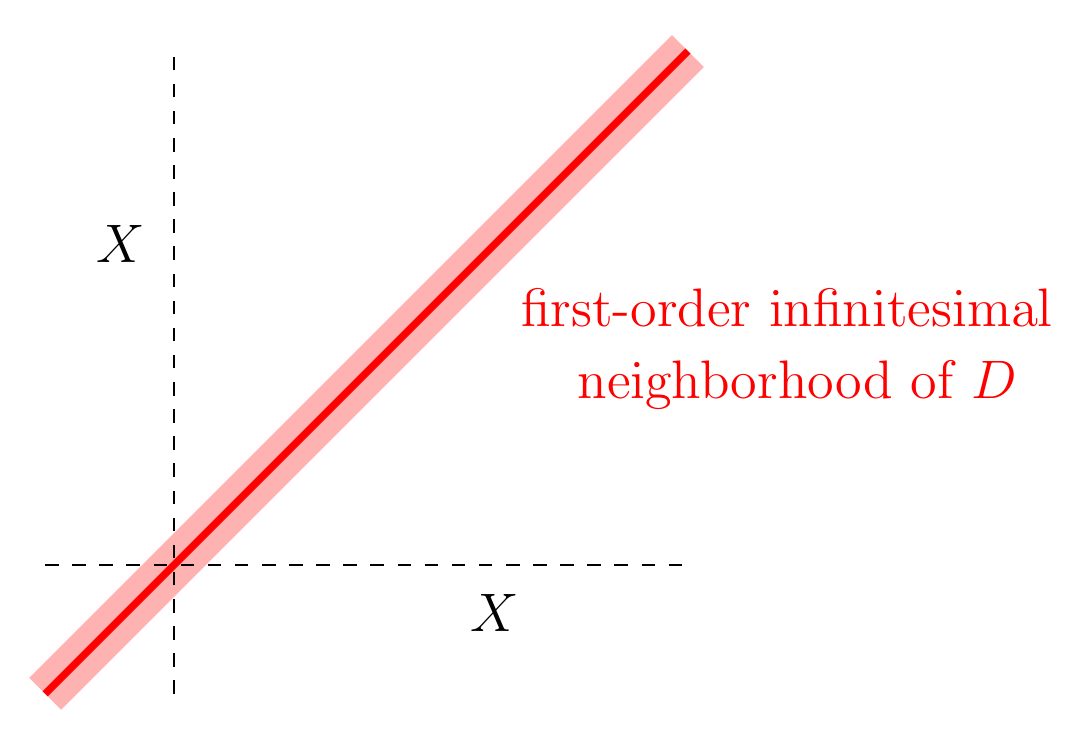## October 12, 2023

### Grothendieck–Galois–Brauer Theory (Part 5)

#### Posted by John BaezLately I’ve been talking about ‘separable commutative algebras’, writing serious articles with actual proofs in them. Now it’s time to relax and reap the rewards! So this time I’ll come out and finally explain the geometrical meaning of separable commutative algebras.

Just so you don’t miss it, I’ll put it in boldface. And in case that’s not good enough, I’ll also say it here! Any commutative algebra $A$ gives an affine scheme $X$ called its spectrum, and $A$ is separable iff $X \times X$ can be separated into the diagonal and the rest!

I’ll explain this better in the article.

Let’s try to get a clearer geometrical picture of what I’ve been talking about lately.

The algebra I’ll be discussing today works for any commutative algebra $A$ over any commutative ring $k$. And so does the geometry! The idea is to think of $A$ as an algebra of functions on some sort of space $X$. Algebraic geometers call this sort of space an affine scheme over $k$, and call $X$ the spectrum of $A$. But don’t let that intimidate you! I’ll draw pictures of very simple cases, like where $X$ is a line.

Since $A$ is the algebra of functions on $X$, $A \otimes A$ is the algebra of functions on $X \times X$. The idea is that given functions $f$ and $g$ on $X$ we get a function $f \otimes g$ on $X \times X$ by

$(f \otimes g)(x,y) = f(x) g(y)$

Notice, there are two different ways to multiply functions in $A \otimes A$ by functions on $A$. One is on the left:

$f (g \otimes h) = f g \otimes h$

This multiplies a function on $X \times X$ by a function that depends only on ‘$x$ coordinate’:

$(f (g \otimes h))(x,y) = f(x) g(x) h(y)$

The other is on the right:

$(g \otimes h)f = g \otimes h f$

This multiplies a function on $X \times X$ by a function that depends only on ‘$y$ coordinate’:

$((g \otimes h)f)(x,y) = g(x) h(y) f(y)$

This fact — that you can multiply functions on $A \otimes A$ by functions in $A$ in two ways, which obey nice associative laws — can be summarized by saying that $A \otimes A$ is an $A,A$-bimodule.

By the way, the term ‘$x$ and $y$ coordinates’ is a bit poetic here: a better thing to say might be ‘first and second variables’. But when $X$ is the real line, my picture of $X \times X$ is this:and in this example, $x$ and $y$ really are just the usual $x$ and $y$ coordinates.

Now, sitting inside $X \times X$ is the diagonal

$D = \{(x,x) \; \vert \; x \in X\} \subseteq X \times X$

This plays a starring role in all that follows, so please visualize it:The functions vanishing on the diagonal form an ideal in $A \otimes A$. Let’s call it $I$. Algebraically, this ideal is precisely the kernel of the multiplication map

$m \colon A \otimes A \to A$

I wish this were more visually obvious to me, but it follows from the proof of Lemma 15.

The quotient ring $(A \otimes A)/I$ is the ring of functions on the diagonal. But of course, the diagonal is isomorphic to $X$ itself. So,

$(A \otimes A)/I \; \cong \; A$

We can dignify this fact by saying we’ve got a short exact sequence of $A,A$-bimodules:

$0 \longrightarrow I \stackrel{i}{\longrightarrow} A \otimes A \stackrel{m}{\longrightarrow} A \to 0$

where $i$ is the inclusion of the ideal $I$ in $A \otimes A$. Here $A$ is an $A,A$-bimodule where the left and right actions of $A$ agree. That makes sense, since on the diagonal we have $x = y$.

In Lemma 16 I pointed out something simple but important: the algebra $A$ is separable iff this short exact sequence splits! Concretely, this means there’s an $A,A$-bimodule homomorphism

$p \colon A \otimes A \to I$

such that $i \circ p$ is the identity. This implies the ideal $I$ is actually a commutative ring, since taking the unit $1 \in A \otimes A$ and applying $p$ to it we get a unit for $I$ — check it out! So, for a separable algebra $A$ we get a way of chopping up $A \otimes A$ like this:

$A \otimes A \cong A \oplus I$

not only as $A,A$-bimodules, but as commutative rings.

This means we can take the ring of functions on $X \otimes X$ and separate it into two parts that are themselves rings. One of them is $I$, the functions that vanish on the diagonal. The other is $A$, which is the functions that vanish off the diagonal.

And now we finally come to the main point:

The geometrical meaning of separability is that we can separate $X \times X$ into two disjoint pieces: the diagonal $D$ and the complement $X \times X - D$.

Yes, the word ‘separable’ actually means you can separate $X \times X$ into two pieces. I thank Tom Leinster for pointing this out to me. Finally the word makes sense.

Most commutative algebras are not separable. An affine scheme $X \times X$ is not usually the coproduct of the diagonal and ‘the rest’. The problem is that ‘the rest’ snuggles up arbitrarily close to the diagonal.

For example, in the world of affine schemes the plane is not the disjoint union of the diagonal line and the rest of the plane. So the pictures I’ve been showing so far do not illustrate the separable case.

Here’s an example of the separable case:Now $X$ is just three points. $X \times X$ is all 9 points shown above. The diagonal $D$ is the red points, and the ‘rest’, $X \times X - D$, is the 6 black points. So $X \times X$ breaks cleanly into the diagonal and the rest.

All this is related to calculus in a great way. $(A \otimes A)/I^2$ consists of functions on $X \times X$ modulo functions that vanish to second order on the diagonal. In our example where $X$ is the real line, $A/I^2$ consists of Taylor series where we expand about the diagonal, like this:

$f(x,y) = f(x,x) + \frac{\partial f(x,y)}{\partial x} (x - y) + \cdots$

but then throw out all the higher order terms! You see, $x - y \in I$ so $(x - y)^2 \in I^2$, and in $A/I^2$ we set it to zero. We’re left with a first-order Taylor series of this form:

$g(x) + h(x) (x - y)$

Since $y - x$ is nonzero in $(A \otimes A)/I^2$ but its square is zero, it acts like an ‘infinitesimal’. This is part of a whole philosophy relating nilpotent elements of rings to infinitesimals.

In general, for any commutative algebra $A$, people call the affine scheme corresponding to $A/I^2$ is the ‘first-order infinitesimal neighborhood of the diagonal’ in $X \times X$. I visualize it sort of like this:It’s hard to draw accurately, because it’s thicker than a line but only infinitesimally thicker. Algebraic geometers often call this sort of infinitesimal thickening ‘nilpotent fuzz’.

Next, what about $I/I^2$? This consists of functions on $X \times X$ that vanish on the diagonal modulo functions that vanish to second order on the diagonal. And these are the same as 1-forms on $X$, since they say to first order how a function changes as you start at a point $(x,x)$ and then start moving to a nearby point $(x,y)$.

But by ‘1-forms’, what I really mean are Kähler differentials, elements of $\Omega^1(A)$. I defined these in Part 3, and Lemma 15 proved that

$I/I^2 \cong \Omega^1(A)$

So, if what I’m saying now seems annoyingly hand-wavy, check that out.

What happens if $A$ is separable? First, the diagonal in $X \times X$ is the same as its own first-order infinitesimal neighborhood. There’s nothing ‘infinitesimally close’ to the diagonal except the diagonal itself! This is easy to believe in our example where $X$ is a finite set of points:But it’s also rigorous math: if $A$ is separable then the ideal $I$ has a multiplicative unit, so $I = I^2$, and

$(A \otimes A)/I^2 \; =\; (A \otimes A)/I \; \cong \; A$

Second, if $A$ is separable there aren’t any nonzero 1-forms on $X$. More precisely:

$\Omega^1(A) \cong I/I^2 \cong 0$

This is a precise way of saying that the affine scheme $X$ is ‘zero-dimensional’.

So, much of what I’ve been doing has a nice geometrical meaning. Atiyah famously claimed:

Algebra is the offer made by the devil to the mathematician. The devil says: “I will give you this powerful machine, it will answer any question you like. All you need to do is give me your soul: give up geometry and you will have this marvellous machine.”

I don’t agree. To me there is insight to be had in algebra as well as geometry: it’s not just a “powerful machine”. But even if you agree with Atiyah, you can cheat the devil. You can compute using algebra, and then go back and interpret what you’ve done using geometry.

By the way, besides algebra and geometry there’s also logic. The diagonal $D \subset X \times X$ corresponds to the predicate $x = y$. If $X$ is the spectrum of a separable algebra, there’s also an affine scheme $X - D \subseteq X \times X$ corresponding to the predicate $x \ne y$, and a version of the ‘law of excluded middle’ holds.

Posted at October 12, 2023 8:48 AM UTC

TrackBack URL for this Entry:   https://golem.ph.utexas.edu/cgi-bin/MT-3.0/dxy-tb.fcgi/3491

### Re: Grothendieck–Galois–Brauer Theory (Part 5)

This has a nice resonance with the Euler characteristic of a manifold as the self intersection of the manifold with itself in the tangent space. This meshes nicely with the infinitesimal neighborhood of the diagonal. This is an algebraic versions of the fact that the normal bundle of the diagonal is the tangent bundle i think.

Posted by: Ana N Mouse on October 13, 2023 1:18 AM | Permalink | Reply to this

### Re: Grothendieck–Galois–Brauer Theory (Part 5)

That’s a great point: there may be a nice algebraic geometry way to define the Euler characteristic of a scheme (or least a variety) in terms of the first-order infinitesimal neighborhood of its diagonal, and this should simplify massively in the separable case.

Posted by: John Baez on October 13, 2023 3:48 PM | Permalink | Reply to this

### Re: Grothendieck–Galois–Brauer Theory (Part 5)

There’s something amiss in the 3rd and 5th displayed equations, in that $h$ doesn’t appear on the right-hand side but presumably should.

I enjoyed the mental image of part of a scheme “snuggling up” to the diagonal.

Posted by: Tom Leinster on October 14, 2023 2:46 PM | Permalink | Reply to this

### Re: Grothendieck–Galois–Brauer Theory (Part 5)

Thanks, fixed!

Jim Dolan had a nice example: explain why the algebra of complex functions on $\mathbb{N}$ is not separable. On the one hand it can’t be separable because there’s a general theorem saying any separable algebra over a field is finite-dimensional. But on the other hand, how can a point snuggle up to the diagonal in this case? $\mathbb{N}$ seems too discrete for much snuggling to occur.

Posted by: John Baez on October 15, 2023 4:09 PM | Permalink | Reply to this

### Re: Grothendieck–Galois–Brauer Theory (Part 5)

I see Jim’s point. Do you have a helpful viewpoint on this example?

Posted by: Tom Leinster on October 16, 2023 11:12 PM | Permalink | Reply to this

### Re: Grothendieck–Galois–Brauer Theory (Part 5)

Aren’t the maximal ideals of $\mathbb{C}^{\mathbb{N}}$ given by ultrafilters on $\mathbb{N}$? If so, then this ring has many more ‘points’ to snuggle up to.

Posted by: Andrew Hubery on October 17, 2023 9:07 AM | Permalink | Reply to this

### Re: Grothendieck–Galois–Brauer Theory (Part 5)

Yes, this is like Jim’s answer to his own puzzle… but probably more precise. This affine scheme is not as discrete as one might think, but all the snuggling happens ‘at infinity’.

Posted by: John Baez on October 17, 2023 12:29 PM | Permalink | Reply to this

### Re: Grothendieck–Galois–Brauer Theory (Part 5)

undefined[object Object]undefined Dr. Baez,

I have studied identity and difference for my entire adult life. It pains me to see so many published statements which are “so close.” I publish this comment only because of your hyperlink to Lawvere’s work. His use of a ring structure in infinitesimal analysis speaks to his paper on the unity of opposites.

The “problem” with algebra is that it presupposes well-construed reflexive equality. A better expression for this is “objectual ontology.” What makes it a better expression is that it can be contrasted with “differential ontology.” Poincare had objected to logicists snd formalists, in part, because 2 can never be 1. Respect this separation, and you can understand “snuggling” much better. This is, after all, what your post has been about.

If you wish to understand “snuggling” in a non-algebraic way, you might begin by looking at the relationship of the 3-circle Venn diagrams and spherical octohedra. The regions of the 3-circle diagram can be correlated with the eight octants. However, one bounded region must be opposed to the unbounded region.

Form a second such diagram with each octant sign convention replaced by its opposite. Now, by the unity of opposites, one may understand what it is to “snuggle” up to the 3-space zero vector.

So much calculation relies upon the use of zero. In turn, emphasis on calculation leads to a focus upon numbers as “objects.” Hence, there is a natural tendency to characterize mathematics in terms of objectual ontology.

This is further reinforced by demands of classical truth as a correspondence theory. Regardless of the rhetorical debates, first-order logic has unique characteristics in this respect. A correspondence theory only makes sense in terms of an objectual ontology. And, differential ontology “breaks” naive conceptions of truth. Category theorists are very familiar with these kinds of debates.

If you would like some motivation for thinking about a spherical octohedron, look at Gauss’ monograph on curved surfaces. It begins by projecting the celestial sphere to a unit sphere. My described construction simply extends the projection to where it “snuggles” the zero vector.

To my knowledge every attempt to use objectual ontology to describe infinitesimals has resulted in a decidable theory of real closed fields. Yet, undecidability is reasserted when this is extended in an unrestricted way to accommodate trigonometric functions (more generally, analytic functions). So, a comparison of logic issues with Gauss’ work ought not be unreasonable.

I note that both prime numbers and trigonometfic functions are mentioned on the Wikipedia page for Venn diagrams.

I note that the ncatlab page about the ubiquity of the number 24 makes no mention of Aristotelian syllogisms. (On that count, recent papers by Vaughn Pratt may expose the category-theoretic relationships to Venn diagrams.)

And, for what this is worth, “snuggling” smacks of physics more than it does of mathematics.

I hope these remarks provide some insights to others, despite being outside of category theory.

Posted by: mitchell smith on October 18, 2023 12:50 PM | Permalink | Reply to this

### Re: Grothendieck–Galois–Brauer Theory (Part 5)

I note that the ncatlab page about the ubiquity of the number 24 makes no mention of Aristotelian syllogisms.

Because that logical form is (a) irrelevant to modern mathematics, and (b) comes in at most eleven forms.

Also, much of what you have been saying is philosophical gibberish - that whole bit about the Venn diagram and the “unity of opposites” has not much to do with anything, and you clearly didn’t realize that “snuggle” meant “limit to” here (it’s just a verb used for color), which is a much more strict thing than just “surround”, or whatever you took it to.

Posted by: NoLongerBreathedIn on October 22, 2023 3:27 PM | Permalink | Reply to this

### Re: Grothendieck–Galois–Brauer Theory (Part 5)

Posted by: John Baez on October 22, 2023 5:52 PM | Permalink | Reply to this

Post a New Comment